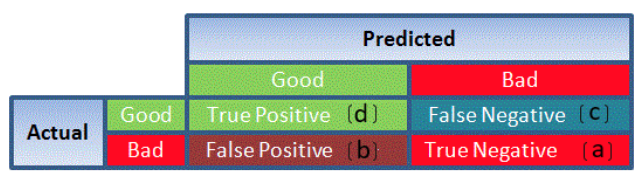# Some common Machine Learning, Statistics and Data Science terms starts with A

## A

[su_table]

### Word ### Description
Accuracy Accuracy is a metric by which one can examine how good is the machine learning model. Let us look at the confusion matrix to understand it in a better way:So, the accuracy is the ratio of correctly predicted classes to the total classes predicted. Here, the accuracy will be:|
|Adam Optimization|The Adam Optimization algorithm is used in training deep learning models. It is an extension to Stochastic Gradient Descent. In this optimization algorithm, running averages of both the gradients and the second moments of the gradients are used. It is used to compute adaptive learning rates for each parameter.

Features:

1. It is computationally efficient and has little memory requirements

2. It is invariant to diagonal rescaling of the gradients

3. Adam works well in practice as compared to other stochastic optimization methods|
|Apache Spark|Apache Spark is an open-source cluster computing framework. Spark can be deployed in a variety of ways, provides native bindings for the Java, Scala, Python, and R programming languages, and supports SQL, streaming data, and machine learning. Some of the key features of Apache Spark are listed below:

4. Speed − Spark helps to run an application in Hadoop cluster, up to 100 times faster in memory, and 10 times faster when running on disk

5. Spark supports popular data science programming languages such as R, Python, and Scala

6. Spark also has a library called MLlIB which includes basic machine learning including classification, regression, and clustering|
|Autoregression|Autoregression is a time series model that uses observations from previous time steps as input to a regression equation to predict the value at the next time step. The autoregressive model specifies that the output variable depends linearly on its own previous values. In this technique input variables are taken as observations at previous time steps, called lag variables.

For example, we can predict the value for the next time step (t+1) given the observations at the last two time steps (t-1 and t-2). As a regression model, this would look as follows:

X(t+1) = b0 + b1X(t-1) + b2X(t-2)

Since the regression model uses data from the same input variable at previous time steps, it is referred to as an autoregression.|